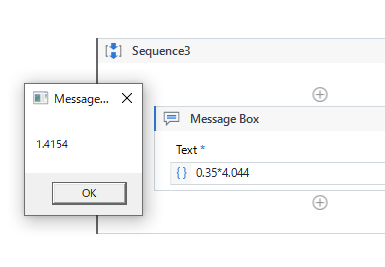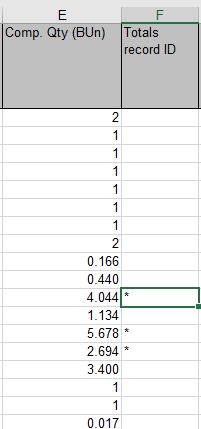# Double interger multiply

Hi I am multiplying two double vale
like 0.354.044 its giving me 0 value
When there is simple integer its giving me correct value
I have used this (COL1
COL2)
Both are double datatype

Hi,

Can you share screenshot of your expression?

It works as the following.Regards,

Can you share the screenshot from your code, is your result being stored in an integer?
If you are multiplying two double, it should return you a value in double variable and should be stored in a double value itself,
Also can you try surrounding your expression in Cdbl(Col1*Col2).

Thanks
Happy Automation!

Used but same things its giving me 0

Can you try to debug and check what values are you getting in col1 and col2?

@Kuldeep_Pandey
Try to convert like this

Convert.ToDouble(“yourcolumn”)
Before multiply convert both your columns values to double. And after that try to multiplyColmumn1 is E
One thing i have noticed u can see in f column there is * if there is * its giving me 0 value
i can’t understand whats the logic
and i have seen double value multiplication is also giving me right value only if * is there in any column its giving 0 after multiplication
My column 2

u

Thankyou but now i see double value is also multiplying now